# Maximum Subarray Problem

In computer science, the maximum subarray problem is the task of finding the contiguous subarray within a one-dimensional array of numbers which has the largest sum. For example, for the sequence of values −2, 1, −3, 4, −1, 2, 1, −5, and 4, the contiguous subarray with the largest sum is 4, −1, 2, 1, with sum 6. (wikipedia.org, 2007).

To solve this problem, we will use Kadane’s Algorithm. It’s essence is in the consistent checking of all positive integers in the array, searching for the contiguous segments and tracking their sums. Every time we get a positive sum we compare it with the current maximum sum, and if it’s bigger, we consider the current sum to be maximal.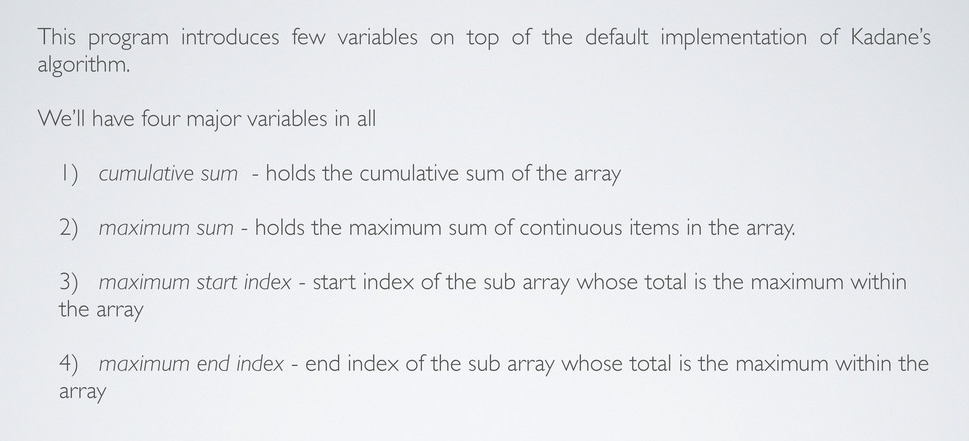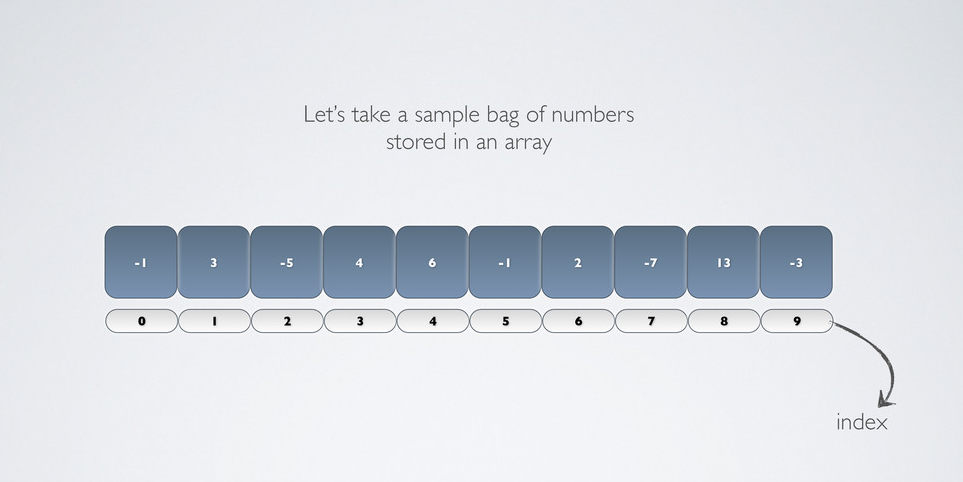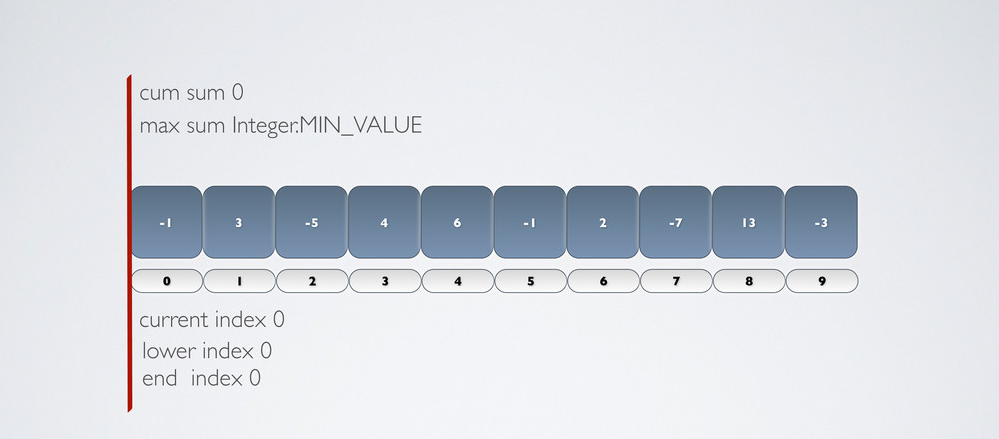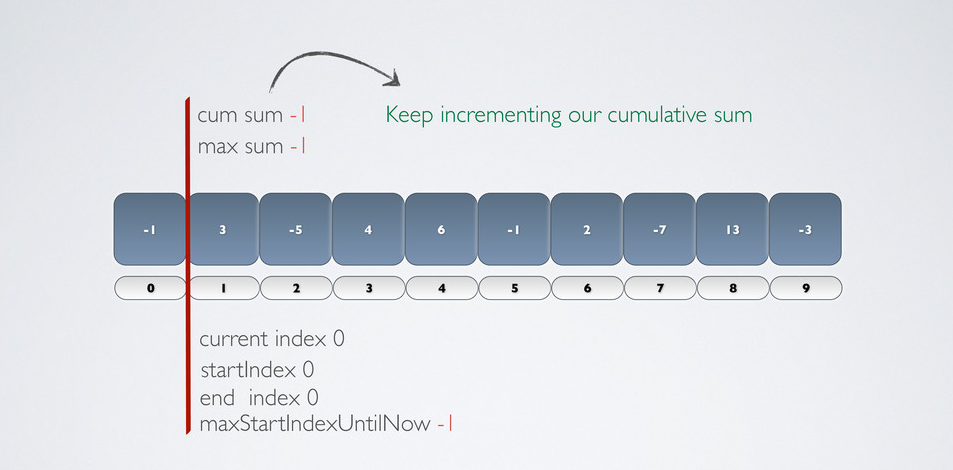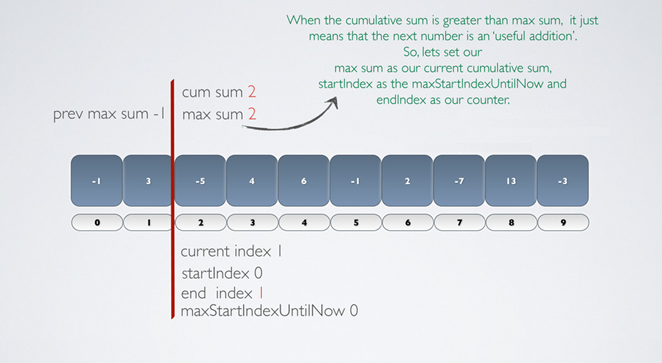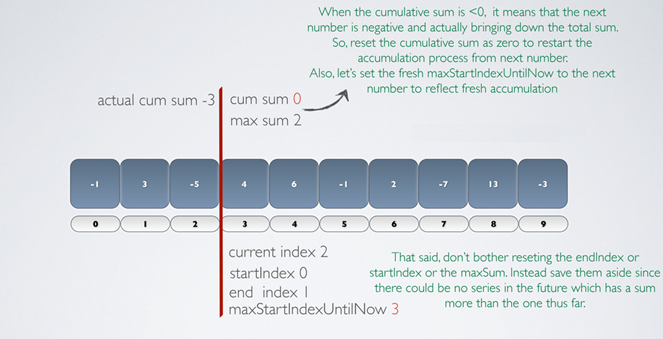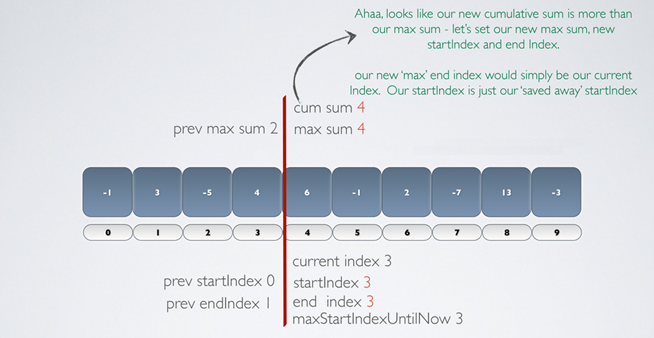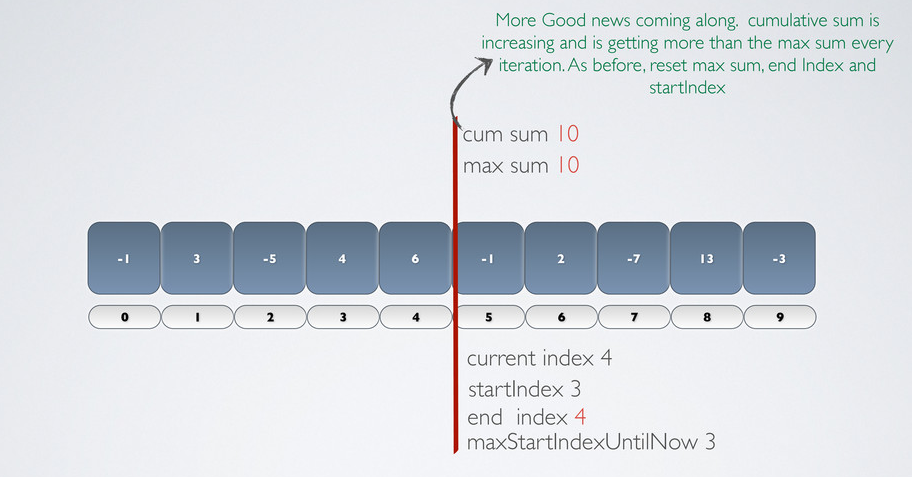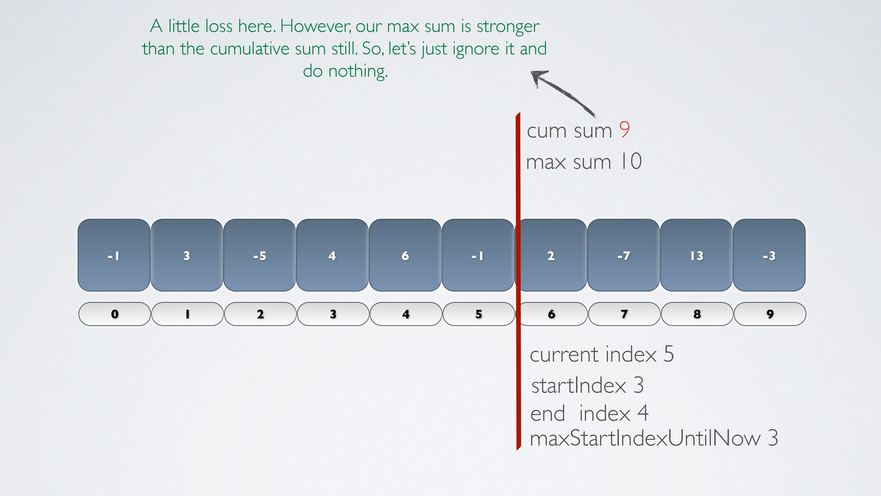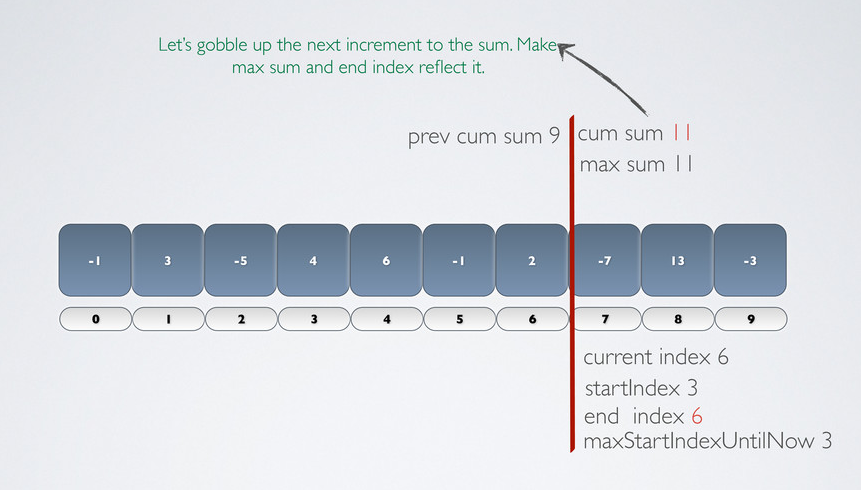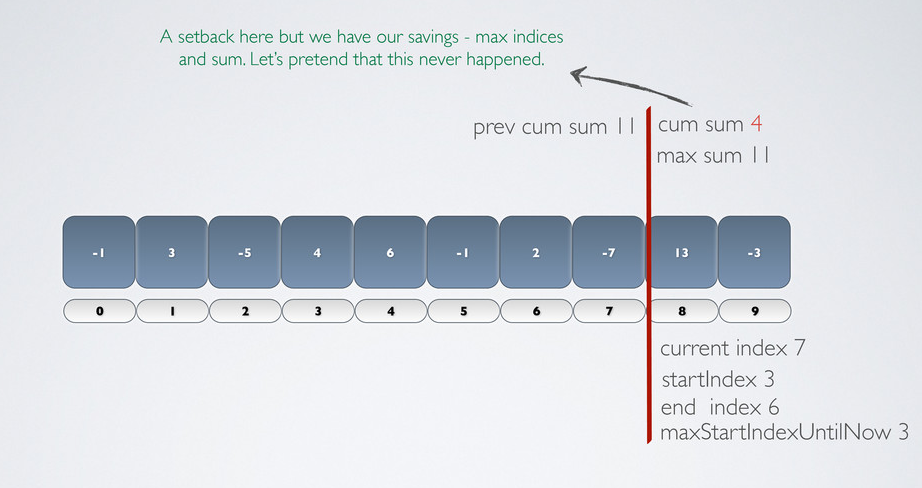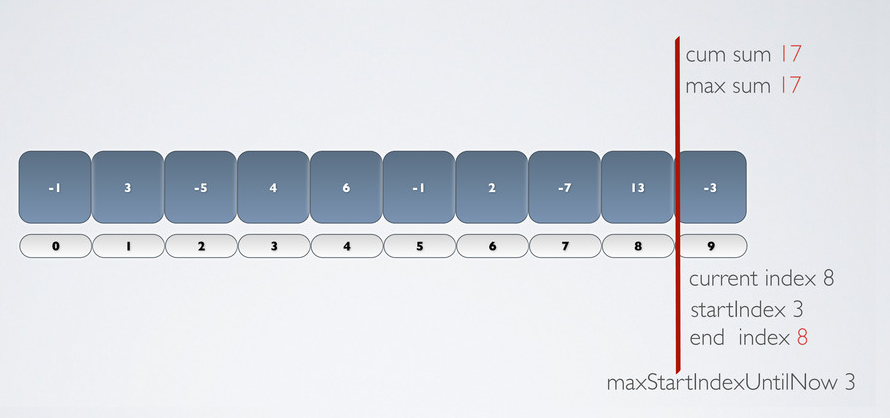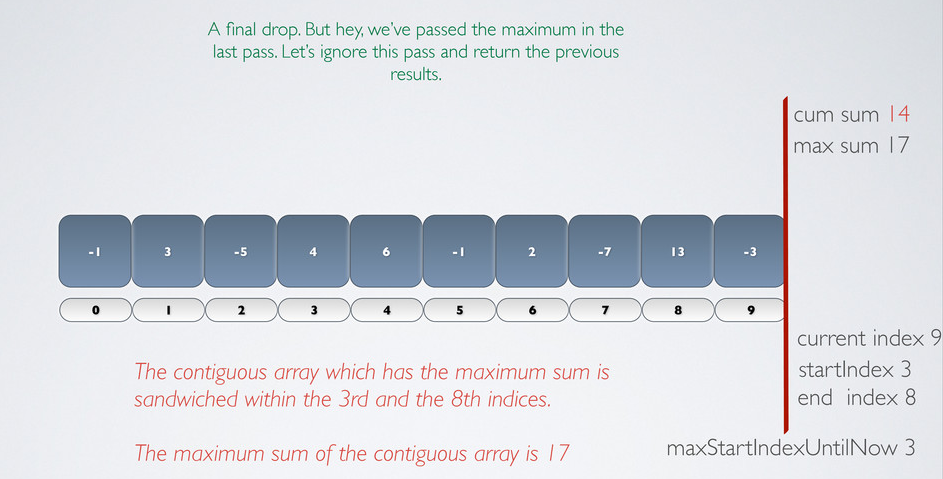Java implementation:

```public class Kadane {

public static void main(String[] args) {
int[] array = { 2, -4, -6, -2, -8, -2, 1, 6, 3, 6 };

searchMaxSub(array);
}

public static void searchMaxSub(int[] inArr) {

int maxStartInd = 0;
int maxEndInd = 0;
int maxSum = Integer.MIN_VALUE;

int aggregateSum = 0;
int tempMaxInd = 0;

for (int currInd = 0; currInd < inArr.length; currInd++) { int arrayItem = inArr[currInd]; aggregateSum += arrayItem; if (aggregateSum>maxSum) {
maxSum = aggregateSum;
maxStartInd = tempMaxInd;
maxEndInd = currInd;
}
else if (aggregateSum<0) {
tempMaxInd = currInd + 1;
aggregateSum = 0;
}
}

System.out.println("Max sum         : " + maxSum);
System.out.println("Max start index : " + maxStartInd);
System.out.println("Max end index   : " + maxEndInd);
}

}
```